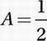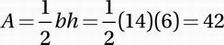# SAT Math Multiple Choice Question 611: Answer and Explanation

### Test Information

Question: 611

11. The graph of the equation y = 2x2 - 16x + 14 intersects the y-axis at point A and the x-axis at points B and C. What is the area of triangle ABC?

• A. 42
• B. 48
• C. 54
• D. 56

Explanation:

Any point that intersects the y-axis has an x-value of 0. So, to find point A, plug in 0 for x and solve for y:

y = 2x2 - 16x + 14

Plug in 0 for x:

y = 2(0)2 - 16(0) + 14 = 14

Any point that intersects the x-axis has a y-value of 0. So, to find points B and C, plug in 0 for y and solve for x:

y = 2x2 - 16x + 14

Substitute 0 for y:

0 = 2x2 - 16x + 14

Divide by 2:

0 = x2 - 8x + 7

Factor:

0 = (x - 7)(x - 1)

Use the Zero Product Property:

x = 7 and x = 1

If we connect these three points, we get a triangle with a height of 14 (from y = 0 to y = 14) and a base of 6 (from x = 1 to x = 7).

Use the triangle area formulabh: